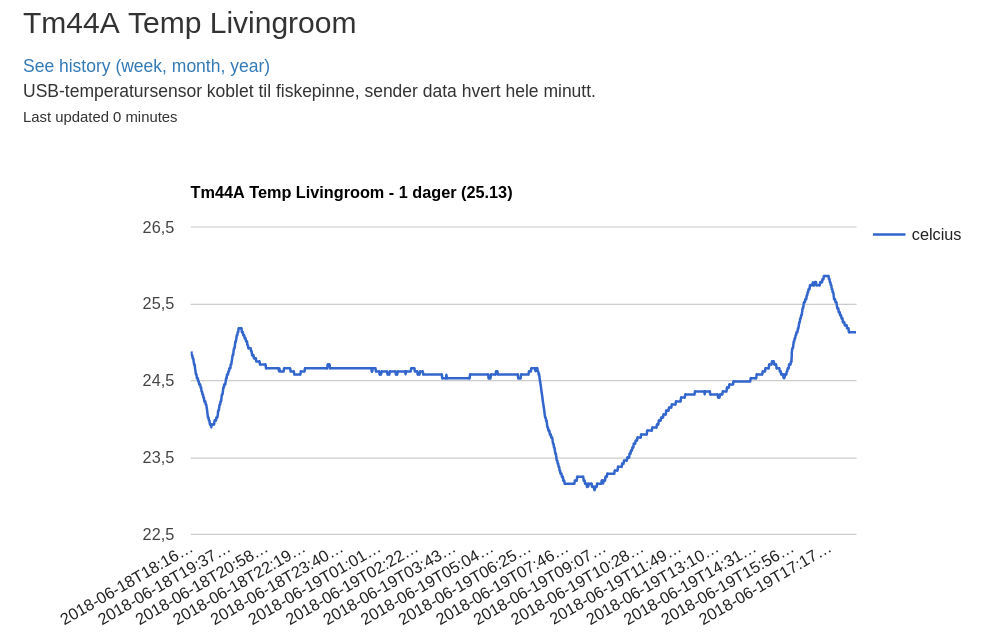# REST statsThis started by me wanting to graph up from some arbitrary data sources, and I wanted to store the data in my postgres database for learning purposes. So I went down the rabbit hole and looked for a statsd-ish services with a Postgres backend, but everything was either a bit to much or a bit to little. So I ended up writing a simple app for my Django project lekvam.no and piggy backed the ubiquitous REST API and the Postgres backend I already had set up.

It is nothing fancy, for gauge measurements; I collect incoming values to the following models. If the gauge's slug (name) is not seen before, its created, so I can dynamically add new measurements from scripts or other sources. The secret is added when the gauge is created and must be present when you want to add data points.

```class Gauge(models.Model):
slug = models.CharField(max_length=100, unique=True)
title = models.CharField(max_length=100)
created_at = models.DateTimeField(auto_now_add=True)
secret = models.TextField(null=True, blank=True)
desc = models.TextField(null=True, blank=True)
unit = models.TextField(null=True, blank=True)
deleted = models.DateTimeField(null=True, blank=True)

class GaugeValue(models.Model):
gauge = models.ForeignKey(Gauge, on_delete=models.CASCADE)
created_at = models.DateTimeField(auto_now_add=True)
value = models.FloatField()
```

I have made a simple dashboard which draws graphs from the latest data on https://lekvam.no/stats/ and you can browse historical data by navigating further. For now, every graph is aggregating over all the values in the given timespan and it is snappy enough. The graphs are made by the Django app graphos which has a collection of straightforward graph types.

A gague can be created, and enriched, with:

```curl -s --get \
--data-urlencode "secret=s3cr3t" \
--data-urlencode "value=\$VALUE" \
https://lekvam.no/stats/gauge/\$SLUG/add
```

The whole code can be found on github.